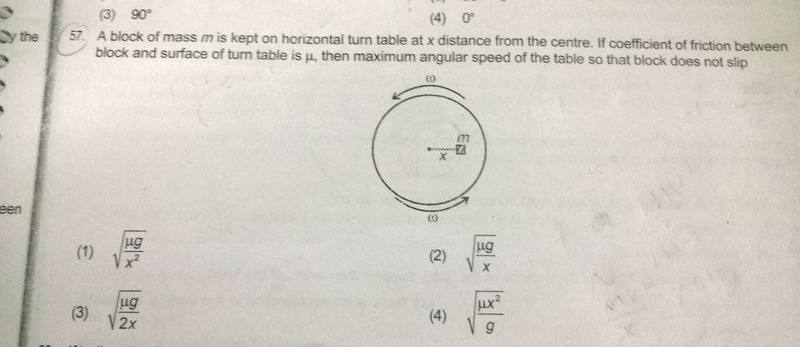# Circular Motion and resultant force

## Homework Statement## Homework Equations

F= mv^2/r
v = angular vel* r

## The Attempt at a Solution

resultant force= ma-frictional force
= mv^2/r - mg*meu
= (angular vel)^2mx - mg*meu
But then how do I get the angular speed when I don;2 know the resultant force?

## Answers and Replies

Doc Al
Mentor
resultant force= ma-frictional force
Only one (horizontal) force acts on the mass.

Only one (horizontal) force acts on the mass.
I don't understand.

Doc Al
Mentor
I don't understand.
Please identify the forces acting on the mass.

Please identify the forces acting on the mass.
There is no centripetal force as the mass is not accelerating.
There is the friction force and centrifugal force

Doc Al
Mentor
There is no centripetal force as the mass is not accelerating.
There is the friction force and centrifugal force
That would be true if viewed from the rotating frame, which requires the inertial centrifugal force. If so, what is the acceleration?

Or you can view it from the usual inertial frame, where the only force would be friction and there would be a centripetal acceleration.

That would be true if viewed from the rotating frame, which requires the inertial centrifugal force. If so, what is the acceleration?

Or you can view it from the usual inertial frame, where the only force would be friction and there would be a centripetal acceleration.

so F=mv^2/r
mv^2/r=N*meu
v*angular vel* m = mg*meu
so angular vel = g*meu/v
as v= angular vel* x
angular vel^2=g*meu/x
so angular vel = (g*meu/x)^(1/2)
Which is the answer, thank you!